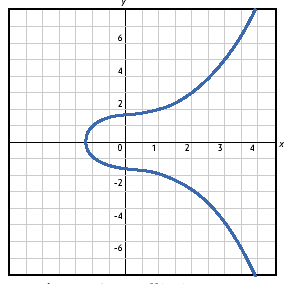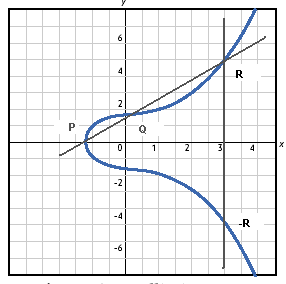## Thursday, February 5, 2009

### [Crypto] Elliptic Curves in Figures

Lately, I got interested in elliptic curve cryptography and have been reading materials on it. All of the cryptographic algorithms rely on the fact that computers are computationally bounded. While this assumption still holds, the bound keeps on pushing up with the advancements in technology. IMO, eventually conventional cryptographic algorithms will be replaced by elliptic curve cryptographic algorithms. I hope the following pictorial tour will give some pointers as to why it will happen and some basics of ECC.

Why does an asymmetric cryptographic system work?(Source: here)

As we increase the key length, the inverse operation exponentially becomes hard while the complexity of the forward operation increases only slowly. It is the hardness of the inverse operation (in conventional systems, the discrete logarithm problem: given y (which is = g^x mod p), g, and p (a large prime), find x) that makes asymmetric cryptography possible. To keep up with the ever increasing computing power, it forces to use longer key lengths.

For RSA 1024 or above. Can we do the job with smaller key while making the inverse operation hard? We need a curve which has a faster exponential growth for the inverse operation.

ECC (Elliptic Curve Cryptography) is the solution.How is it possible? Both CC (Conventional Cryptography) and ECC use groups. The difference is how the group and its operation for ECC is defined. In CC, we use multiplicative groups of integer modulo p. In ECC, we actually use elliptic curves!

How does an EC look like? It has the form y^2 = x^3 + ax + b.The points on the EC are the members of the group. Actually it has one extra member; it i the identity element and denoted by infinity. Addition of points is the group operation. But how exactly do we add points?If P and Q are two on the EC, P + Q = -R where -R is the third point on the curve provided that the line passing through P and Q is not vertical. If it is vertical, there can be at most two points on the EC. Those two points have the form R and -R. For example R = (x, y) and -R = (x, -y).

What if we add a point to itself?P + P = -R (draw a tangent, if it is not vertical, it will intersect the EC at one more point (which is R). The point of reflection is -R)

Point multiplication is what we use to build a asymmetric cryto system: kP = P + P + ... + P (k times). Even though it is difficult to visualize graphically, there are efficient algorithms to calculate this. What is the inverse here? Given the points P and Q on E, find k such that Q = kP. It is a hard problem and is the equivalent of discrete logarithm problem in ECC.

For example, here is how ElGamal public key cryptosystem works.

Fix an elliptic curve E modulo p and select a point Q of large order on E (Like fixing a large prime p and selecting a primitive root 1 < g < p in Classical ElGamal (CE for short)). (Just like g, Q is also public)

Each user has a private key. Alice selects a secret 0 < a_A < p (same as in CE).

Alice publishes her public key b_A = a_A.Q (Just like b_A = g^a_A in CE).

Now Bob wants to send a secret message M to Alice (this message is actually embedded to a point in E). Bob selects a random k such that 0 < k < p and sends Alice the following pair.

C = (kQ, kb_A + M) (Like C = (g^k, M.b_A^k) in CE)

Alice finds M from C.

(k.b_A + M) - (k.Q)a_A = (k.a_A.Q + M) - k.a_A.Q = M (Like M.b_A^k/g^k^b_A = M in CE).

If an eavesdropper to find M without knowing a_A (Alice's secret), she needs to solve the discrete logarithm problem!

References: here and Wagstaff's Cryptanalysis book

#### 1 comment:

Blogger said...

Need To Increase Your ClickBank Banner Commissions And Traffic?

Bannerizer makes it easy for you to promote ClickBank products using banners, simply visit Bannerizer, and grab the banner codes for your picked ClickBank products or use the Universal ClickBank Banner Rotator Tool to promote all of the ClickBank products.